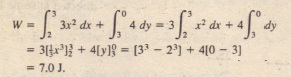# Sample Problem Kinetic Energy And Work

Sample Problem Kinetic Energy And Work

Force with x in meters, acts on a particle, changing only the kinetic energy of the particle. How much work is done on the particle as it moves from coordinates (2 m, 3 m) to (3 m, 0 m)? Does the speed of the particle increase, decrease, or remain the same?

SOLUTION: The Key Idea here is that the force is a variable force because its x component depends on the value of x. Thus, we cannot use find the work done. Instead, we must use.The positive result means that energy is transferred to the particle by force F. Thus, the kinetic energy of the particle increases, and so must its speed.

Share This